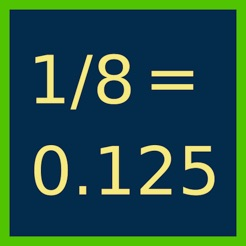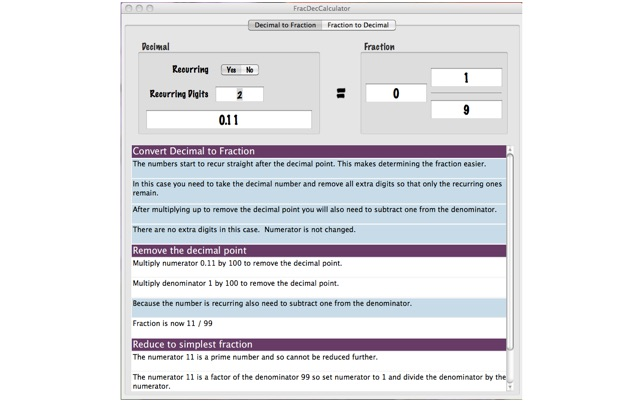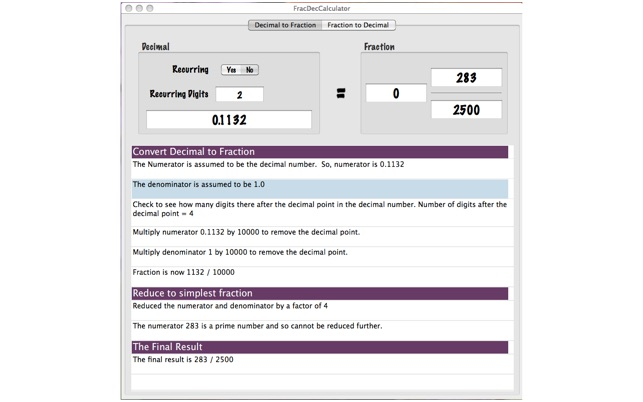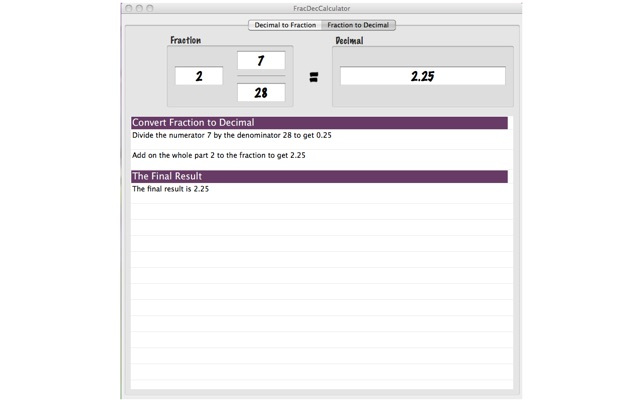## Screenshots

•••## Description

Do you need to convert fractions into decimals or decimals into fractions? Can't quite remember how to do it? Well this App is for you. Not only does it do the calculations for you but it also provides comprehensive explanations as to how it was done so you can understand how the answer was arrived at helping you learn. Some calculations are easy but others such as converting a recurring decimal into a fraction are not. This App explains how it is done step by step.

Fraction to Decimal:

The App can take a fraction and convert it into its decimal equivalent. Below the answer is the workings to show how the answer was arrived at. If the answer is extremely long then chances are that the number is recurring. If the digits after the decimal point start repeating then the number is probably recurring.

Decimal to Fraction:

The App can also take a decimal and convert it into its equivalent fraction. Below the answer is the workings to show how the answer was arrived at. The decimal number can either be specified as recurring or not and if it is how many digits are recurring.

When specifying the number of recurring digits you specify the number from the right hand side. So, to specify 0.125252525.... where the '25' part recurs you would put in 0.125, recurring is 'Yes' and number of recurring digits are 2 - meaning that 2 digits from the right hand side recur (i.e. the '25' part of the decimal).

Some examples,

0.3 not recurring is 3/10

0.3 with one digit recurring is 1/3 (specify recurring Yes and recurring digits 1)

0.311 not recurring is 311/1000

0.301 with one digit recurring is 271/900 (specify recurring Yes and recurring digits 1)

0.301 with two digits recurring is 149/495 (specify recurring Yes and recurring digits 2)

0.142857142857 with six digits recurring is 1/7 (specify recurring Yes and recurring digits 6)

In the final example the 6 recurring digits are specified twice but this does not matter to the App. The excess digits are carefully removed and the calculation done with 0.142857

## Ratings and Reviews

3.5 out of 5
2 Ratings

2 Ratings

aqh2013 ,

### Awesome!

This app does exactly what is stated in the description. This handy app explains step by step how this process works and is a wonderful tool for anyone that needs to learn or re-learn these concepts. Great job!

## Information

Seller
Jim Chapple
Size
154.2 KB
Category
Education
Compatibility

OS X 10.6 or later

Languages

English

Age Rating
Rated 4+
Copyright
© 2011 Essence Computing Ltd
Price
\$0.99

## Supports

•